# Java Program to Check Whether a Number is Prime or Not

In this tutorial you will learn about the Java Program to Check Whether a Number is Prime or Not and its application with practical example.

In this tutorial, we will learn to create a Java program that will Check Whether a Number is Prime or not using Java programming.

## Prerequisites

Before starting with this tutorial we assume that you are best aware of the following Java programming topics:

• Java Operators.
• Basic Input and Output
• Class and Object.
• Basic Java programming.
• If-else Statements.
• Loop in Java.

## What Is Prime number?

Any positive Number which is bigger than 1 and it can only  divisible by itself and by 1 and it has maximum of factors is two is called as Prime number.Rather we can say that a prime number is a number which only divided by him or 1.

Example:->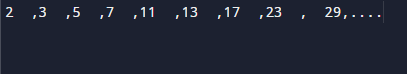Note : 2  is the only positive(even)  number and smallest integer prime number.

## Java Program to Check Whether a Number is Prime or Not

In our program we will find that given number by user is Prime number or not using while loop.
Firstly we declare required header file and variable and also initiates required values in variable. Next we take value from user at run time and then after we will find that the given value is prime number or not.

## Output

Prime NumberNot  a Prime Number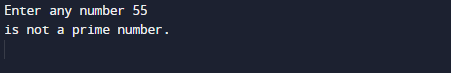In the above program, we have first declared and initialized a set variables required in the program.

• number = number to hold given value.
• count = count for number divisibility occurrence.
• i= for iteration of a loop.

In the next statement user will prompted to enter a number which will be assigned to variable ‘number’. Now loop start from 2 to a given number.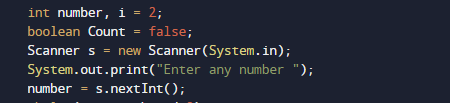In ever while condition we will check ‘number’ is completely divisible by ‘i’ or not.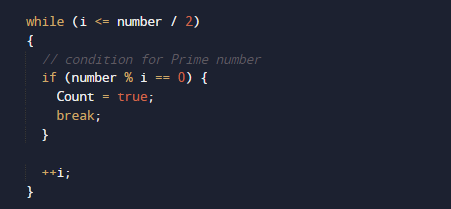Then we check if number that divides completely we set Count Value to ‘true’.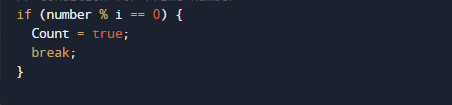or else the value of the value of count default is set to False

So , if given number is Prime after the end of loop the value of count=true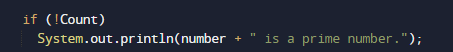however if given number is not the value of count is false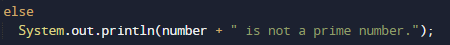So , we will check that if number is a prime number then the value of count must be true.

In this tutorial we have learn about the Java Program to Check Whether a Number is Prime or Not and its application with practical example. I hope you will like this tutorial.